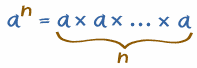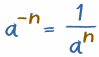# 指数82里，"2"的意思是把两个 8 相乘，

### 例子：53 = 5 × 5 × 5 = 125

• 用文字来说： 53 也可以说成 "5 的 3次方" 或 "5 的立方"

### 例子：24 = 2 × 2 × 2 × 2 = 16

• 用字来说： 24 也可以说成 "2 的 4次方"

## 一般来说

 an告诉你去把a相乘。 总共有n个a相乘：## 其他写法

• 2^4 = 2 × 2 × 2 × 2 = 16

## 负指数

5-3 可以这样计算：

1 ÷ (5 × 5 × 5) = 1/53 = 1/125 = 0.008

## 一般来说刚才的例子是计算负指数的简易方法： 先计算正指数（an） 然后计算倒数（就是1/an）

4-2 = 1 / 42 = 1/16 = 0.0625
10-3 = 1 / 103 = 1/1,000 = 0.001
(−2)-3 = 1 / (−2)3 = 1/(−8) = -0.125

## 如果指数是 1 或 0 呢？

 1 若指数是 1，答案就是数自己(例子 91 = 9) 0 若指数是 0，答案是 1 （例子 90 = 1） 那 00 呢？它可以是 1 或 0，所以大家叫它为"不确定的"。

## 有道理

.. etc..52 1 × 5 × 5 25
51 1 × 5 5
50 1 1
5-1 1 ÷ 5 0.2
5-2 1 ÷ 5 ÷ 5 0.04
。。。等等。。。

## 分组时要小心

 有（）： (−2)2 = (−2) × (−2) = 4 无（）： −22 = −(22) = − (2 × 2) = −4

 有（）： (ab)2 = ab × ab 无（）： ab2 = a × (b)2 = a × b × b